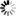Welcome to Central Library, SUST
Normal view

# Mathematics of Fuzzy Sets and Fuzzy Logic [electronic resource] / by Barnabas Bede.

Material type:TextSeries: Studies in Fuzziness and Soft Computing ; 295Publisher: Berlin, Heidelberg : Springer Berlin Heidelberg : Imprint: Springer, 2013Description: XII, 276 p. online resourceContent type:
• text
Media type:
• computer
Carrier type:
• online resource
ISBN:
• 9783642352218
Additional physical formats: Printed edition:: No titleDDC classification:
• 006.3 23
LOC classification:
• Q342
Online resources:
Contents:
1 Fuzzy Sets -- 2 Fuzzy Set-Theoretic Operations -- 3 Fuzzy Relations -- 4 Fuzzy numbers -- 5 Fuzzy Arithmetic -- 6 Fuzzy Inference -- 7 Single Input Single Output Fuzzy Systems -- 8 Fuzzy Analysis -- 9 Fuzzy Differential Equations -- 10 Extensions of Fuzzy Set Theory -- 11 Possibility Theory -- 12 Fuzzy Clustering -- 13 Fuzzy Transform -- 14 Arti cial Neural Networks and Neuro-Fuzzy Systems.
Summary: This book presents a mathematically-based introduction into the fascinating topic of Fuzzy Sets and Fuzzy Logic and might be used as textbook at both undergraduate and graduate levels and also as reference guide for mathematician, scientists or engineers who would like to get an insight into Fuzzy Logic.   Fuzzy Sets have been introduced by Lotfi Zadeh in 1965 and since then, they have been used in many applications. As a consequence, there is a vast literature on the practical applications of fuzzy sets, while theory has a more modest coverage. The main purpose of the present book is to reduce this gap by providing a theoretical introduction into Fuzzy Sets based on Mathematical Analysis and Approximation Theory. Well-known applications, as for example fuzzy control, are also discussed in this book and placed on new ground, a theoretical foundation. Moreover, a few advanced chapters and several new results are included. These comprise, among others, a new systematic and constructive approach for fuzzy inference systems of Mamdani and Takagi-Sugeno types, that investigates their approximation capability by providing new error estimates.  .
Tags from this library: No tags from this library for this title.
Star ratingsAverage rating: 0.0 (0 votes)
No physical items for this record

1 Fuzzy Sets -- 2 Fuzzy Set-Theoretic Operations -- 3 Fuzzy Relations -- 4 Fuzzy numbers -- 5 Fuzzy Arithmetic -- 6 Fuzzy Inference -- 7 Single Input Single Output Fuzzy Systems -- 8 Fuzzy Analysis -- 9 Fuzzy Differential Equations -- 10 Extensions of Fuzzy Set Theory -- 11 Possibility Theory -- 12 Fuzzy Clustering -- 13 Fuzzy Transform -- 14 Arti cial Neural Networks and Neuro-Fuzzy Systems.

This book presents a mathematically-based introduction into the fascinating topic of Fuzzy Sets and Fuzzy Logic and might be used as textbook at both undergraduate and graduate levels and also as reference guide for mathematician, scientists or engineers who would like to get an insight into Fuzzy Logic.   Fuzzy Sets have been introduced by Lotfi Zadeh in 1965 and since then, they have been used in many applications. As a consequence, there is a vast literature on the practical applications of fuzzy sets, while theory has a more modest coverage. The main purpose of the present book is to reduce this gap by providing a theoretical introduction into Fuzzy Sets based on Mathematical Analysis and Approximation Theory. Well-known applications, as for example fuzzy control, are also discussed in this book and placed on new ground, a theoretical foundation. Moreover, a few advanced chapters and several new results are included. These comprise, among others, a new systematic and constructive approach for fuzzy inference systems of Mamdani and Takagi-Sugeno types, that investigates their approximation capability by providing new error estimates.  .

There are no comments on this title.